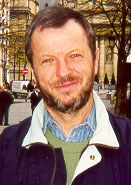## Vladimir I. Piterbarg

### Head of Laboratory

Date of Birth: August, 01, 1945
Degrees: Candidate of Sciences (PhD), 1972, Moscow State University
Doctor of Physics and Mathematics, 1984, Moscow State University,
Professor, 1990, State Education Committee of the USSR.

### Research activities:

1. Asymptotic analysis of random processes and fields. Limit theorems for large deviations. General methods of large excursions and deviations of Gaussian processes and fields analysis as well as methods of long time analysis have been developed. Main results obtained by the methods are:
• Evaluation of exact asymptotic behavior of large excursion probabilities for a wide class of Gaussian processes and fields including second order approximations and asymptotic expansions; \item development of necessary and sufficient conditions in Poisson limit theorem for large deviations of Gaussian discrete time processes;
• central limit theorem for general additive functionals of Gaussian stationary processes in adapted to Gaussian distributions assumptions has been proved.
2. ### Mathematical Statistics and its Applications

• New approach to testing stationarity hypothesis for near nonstationary time series has been advanced with applications to hyperinflation processes modeling.
• Asymptotic behavior of a class of Kolmogorov-Smirnov multidimensional statistics has been evaluated to receive simple approximation formulas for Kolmogorov-Smirnov multidimensional goodness of fit tests.
• Large deviations of kernel density estimators in both one dimensional and multidimensional cases in uniform metrics has been investigated and exact asymptotic behaviors have been evaluated.
3. ### Applications in biology:

• New method of investigation of a homologity of biopolymer sequences based on diffusion approximations has been developed. A limit theorem and approximation formulas for matching probabilities has been proved.
4. ### Applications in engineering design and precision technologies:

• Dimension chains analysis for ball bearing has been performed.
5. ### Other topics of interest

Statistical methods in Reliability Theory and Safety Control. Probabilistic methods in the fatigue of materials.

### Monograph and other main publications

• Lectures in the Theory of Gaussian Processes. Moscow Univ. Press, 1986, 88pp
• Asymptotic Methods in the Theory of Gaussian Processes. Moscow Univ. Press, 1988, 176pp
• Asymptotic Methods in the Theory of Gaussian Processes. 1995, 205pp, Rewritten and Completed Issue, American Mathematical Society, ser. Translations of Mathematical Monographs, Vol. 148.
• On maximum distribution for a Gaussian field with constant variance and indexed by a smooth manifold. (in coop. T.L.Mikhaleva) Theory of Probab. and Appl., 41, 1996, No 2
• High excursion for nonstationary generalized Chi-square processes. Stochastic Processes and Appl, 1994, vol. 53, No 2, 307-337.
• Laplace method for random elements in Banakh spaces. Russian mathematical surveys, 1995, No. 6, 1-102, (in coop. with V. Fatalov)
• High deviations for multidimensional stationary Gaussian process with independent components. in: Problems of Stability of Stochastic Models. V.M. Zolotarev, ed. Moscow-Utrehrt, TVP-VSP, 1994, 197-230.
• Central limit theorem for wave-functionals of Gaussian processes. accepted by Advances of Applied Probability, 1998, (in coop. with I. Rychlik)
• Rice's method for Gaussian random fields. Fundamental and Applied Mathematics, 1996, vol.2, No 1, 20 pp. (in Russian)
• On limit distribution of the quadratic deviation of a kernel estimator of density function in the deconvolution problem. Mathematical Methods of Statistics, Alerton Press, 1993, {\bf 1}, 147-164 (in coop. M.Ya. Penskaya).
• Homogeneity testing of two samples: Gaussian field on a sphere. Mathematical Methods of Statistics, 1993, vol. 2, pp. 147-164 (in coop. Yu.Tyurin)
• High level excursions of Gaussian fields and the weakly optimal choice of the smoothing parameter.I. Mathematical Methods of Statistics, Alerton Press, 1995, No 4, (in coop. with V. Konakov)
• High level excursions of Gaussian fields and the weakly optimal choice of the smoothing parameter.II. Mathematical Methods of Statistics, Alerton Press, 1996, No 4, (in coop. with V. Konakov)
• A Multidimensional Rank Correlation: a Gaussian Field on a Torus. University Of North Carolina at Chapel Hill, Center for Stochastic Processes, Techn. Rep. No. 482, July, 1996, 1-18 (in coop. Yu.Tyurin)
• On large jumps of a random walk. Theory Probability and Its Appl. 1991, {\bf 36}, 1991, 50-63.
• On the distribution of the maximum similarity score for fragments of two random sequences. in: Mathematical Methods of Analysis of biopolymer sequences. Ed. Simon Gindikin, DIMACS Series in Discrete Mathematics and Theoretical Computer Science, 1992, Volume 8, 11-18.

Back to Staff of Laboratory of Probability page